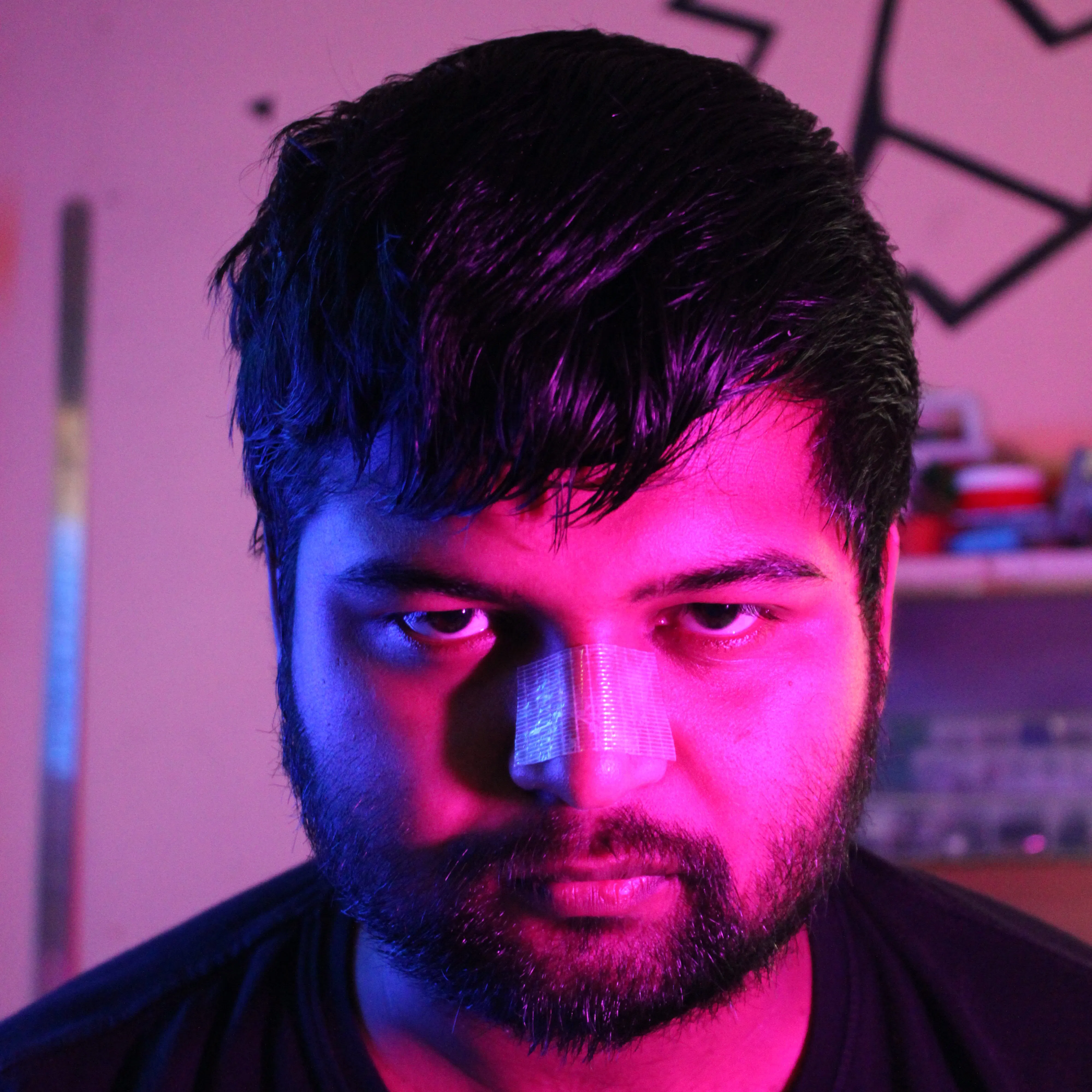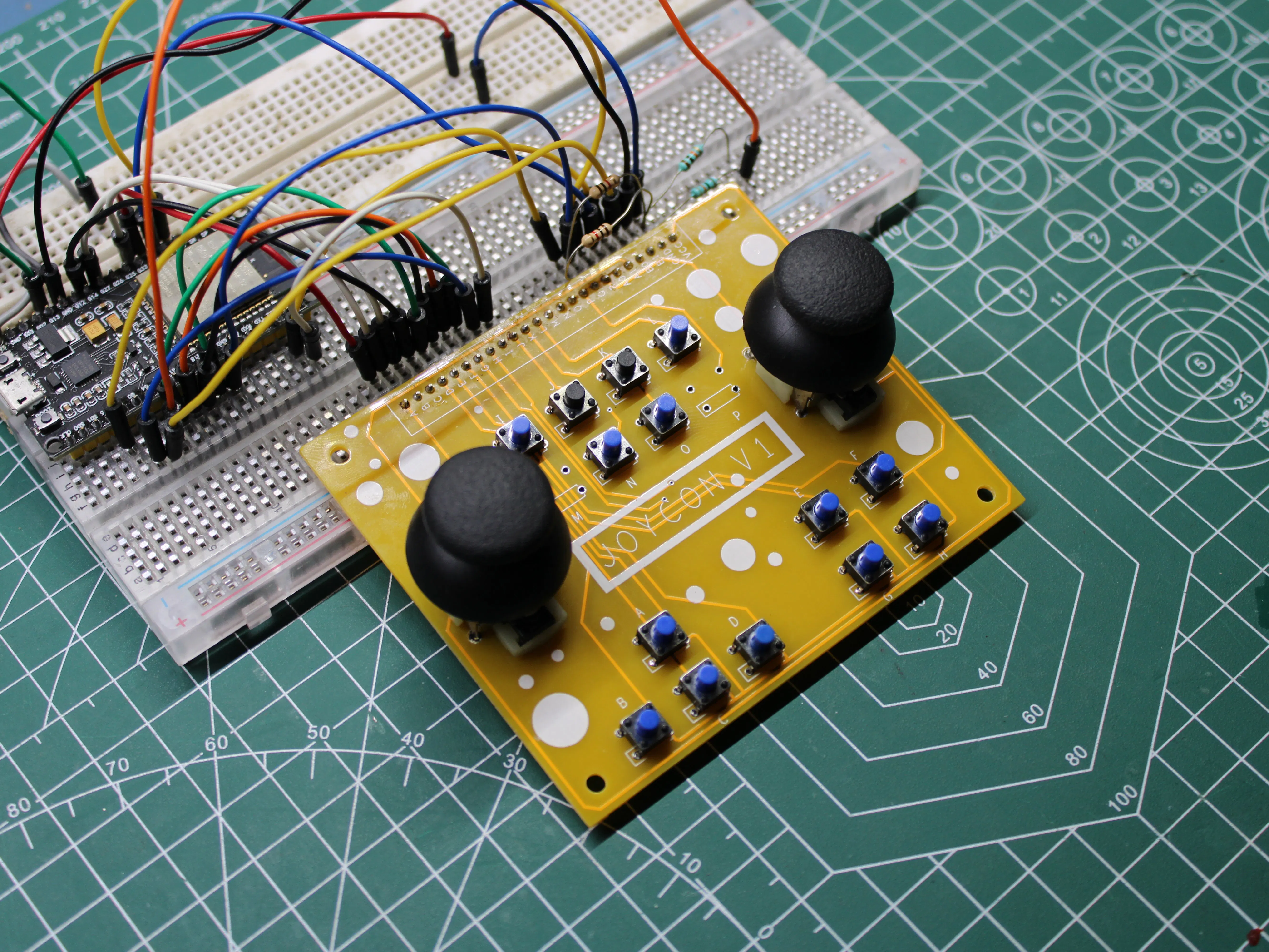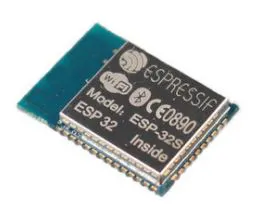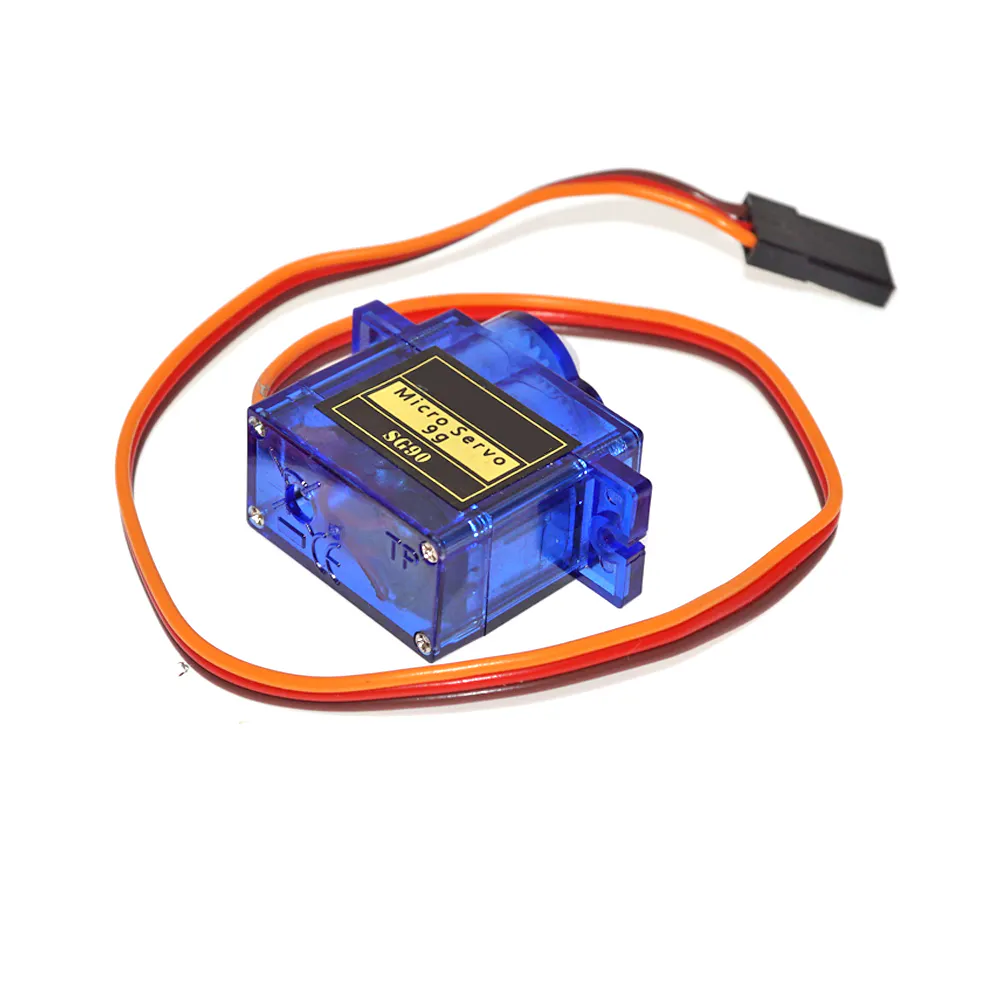# JOYCON Button and Analog Stick Board

Joycon is a free-to-use button board that we can use for making XYZ stuff that requires the use of buttons or an analog joystick.

BeginnerFull instructions provided1 hour233## Things used in this project

### Hardware componentsEspressif ESP32S
×1PCBWay Custom PCB
×1Arduino Nano R3
×1SG90 Micro-servo motor
×1LED (generic)
×1SparkFun Pushbutton switch 12mm
×1

### Software apps and online servicesArduino IDE

## Code

### code

C/C++
```#include <BleGamepad.h>
#include <FastLED.h>

CRGB leds;

// TRIGGER BUTTONS
#define LT 2
#define RT 23

// LEFT-JOYSTICK
#define LH 34
#define LV 35
#define LS 15

// RIGHT-JOYSTICK
#define RH 22    //39
#define RV 0     //36
#define RS 19

#define L1 32
#define L2 33
#define L3 27
#define L4 14

#define R1 18
#define R2 5
#define R3 17
#define R4 16

// BATTERY VOLTAGE

// NEOPIXEL
#define DATA_PIN 13

// PERIFERALS
//  #define MIC 25
//  #define SPEAKER 26

int buttons = {32, 33, 27, 14, 18, 5, 17, 16};

int period = 1000;
unsigned long time_now = 0;

const int numberOfPotSamples = 5;     // Number of pot samples to take (to smooth the values)
const int delayBetweenSamples = 2;    // Delay in milliseconds between pot samples
const int delayBetweenHIDReports = 5; // Additional delay in milliseconds between HID reports
const int debounceDelay = 10;        // Delay in milliseconds between button press

int previousButton1State = HIGH;
int previousButton2State = HIGH;
int previousButton3State = HIGH;
int previousButton4State = HIGH;

void setup() {
Serial.begin(115200);
FastLED.setBrightness(64);
for (int i = 0; i < 8; i++) {
pinMode(buttons[i], INPUT_PULLUP);
}
pinMode(LT, INPUT_PULLUP);
pinMode(RT, INPUT_PULLUP);
pinMode(LS, INPUT_PULLUP);
pinMode(RS, INPUT_PULLUP);
Serial.println("Starting BLE work!");

leds = CRGB::Blue;
leds = CRGB::Blue;
FastLED.show();
delay(200);
leds = CRGB::Blue;
leds = CRGB::Blue;
FastLED.show();
delay(300);
leds = CRGB::Blue;
leds = CRGB::Blue;
FastLED.show();
delay(1000);
FastLED.clear();
FastLED.show();
}

void loop() {
for (int i = 0; i < 6; i++) {
leds[i] = CHSV( HUE_GREEN, 255, 64);
}
FastLED.show();
while (millis() > time_now + period) {
Serial.println("Checking Battery Level");
batteryLevel();
time_now = millis();
}

if (currentButton1State != previousButton1State) {
if (currentButton1State == LOW)
else
}
previousButton1State = currentButton1State;

if (currentButton2State != previousButton2State) {
if (currentButton2State == LOW)
else
}
previousButton2State = currentButton2State;

if (currentButton3State != previousButton3State) {
if (currentButton3State == LOW)
else
}
previousButton3State = currentButton3State;

if (currentButton4State != previousButton4State) {
if (currentButton4State == LOW)
else
}
previousButton4State = currentButton4State;

Serial.println("Left Joystick");
delay(debounceDelay);
}
Serial.println("Right Joystick");
delay(debounceDelay);
}

Serial.println("Left trigger");
delay(debounceDelay);
}
Serial.println("Right trigger");
delay(debounceDelay);
}

int potValues[numberOfPotSamples];
for (int i = 0 ; i < numberOfPotSamples ; i++) {
delay(delayBetweenSamples);
}
int potValue = 0;
for (int i = 0 ; i < numberOfPotSamples ; i++) {
potValue += potValues[i];
}
potValue = potValue / numberOfPotSamples;
int adjustedValue = map(potValue, 0, 4095, 127, -127);

int potValues2[numberOfPotSamples];
for (int i = 0 ; i < numberOfPotSamples ; i++) {
delay(delayBetweenSamples);
}
int potValue2 = 0;
for (int i = 0 ; i < numberOfPotSamples ; i++) {
potValue2 += potValues2[i];
}
potValue2 = potValue2 / numberOfPotSamples;
int adjustedValue2 = map(potValue2, 0, 4095, 127, -127);

int potValues3[numberOfPotSamples];
for (int i = 0 ; i < numberOfPotSamples ; i++) {
delay(delayBetweenSamples);
}
int potValue3 = 0;
for (int i = 0 ; i < numberOfPotSamples ; i++) {
potValue3 += potValues3[i];
}
potValue3 = potValue3 / numberOfPotSamples;
int adjustedValue3 = map(potValue3, 0, 4095, 255, 0);

int potValues4[numberOfPotSamples];
for (int i = 0 ; i < numberOfPotSamples ; i++) {
delay(delayBetweenSamples);
}
int potValue4 = 0;
for (int i = 0 ; i < numberOfPotSamples ; i++) {
potValue4 += potValues4[i];
}
potValue4 = potValue4 / numberOfPotSamples;
int adjustedValue4 = map(potValue4, 0, 4095, 255, 0);
/*
Serial.print(" || ");
Serial.print(" || ");
Serial.print(" || ");
*/
delay(delayBetweenHIDReports);

}
}

void batteryLevel() {
float voltage = sensorValue * (5.12 / 4095.0);
Serial.print(voltage);
Serial.print("V ||");
int percentage = (voltage / 4.2) * 100;
Serial.print(percentage);
Serial.println("%");
if (percentage < 60) {
Serial.println("LOW battery");
for (uint8_t i = 30; i < 220; i++) {
for (int j = 0; j < 6; j++) {
leds[j] = CHSV( HUE_RED, 255, i);
}
FastLED.show();
delay(15);
}
for (uint8_t i = 220; i > 30; i--) {
for (int j = 0; j < 6; j++) {
leds[j] = CHSV( HUE_RED, 255, i);
}
FastLED.show();
delay(15);
}
}
}
```

## Credits

### Arnov Sharma

33 projects • 266 followers